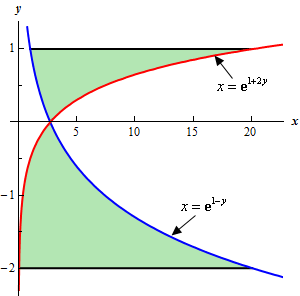Paul's Online Notes
Home / Calculus I / Applications of Integrals / Area Between Curves
Show Mobile Notice Show All Notes Hide All Notes
Mobile Notice
You appear to be on a device with a "narrow" screen width (i.e. you are probably on a mobile phone). Due to the nature of the mathematics on this site it is best views in landscape mode. If your device is not in landscape mode many of the equations will run off the side of your device (should be able to scroll to see them) and some of the menu items will be cut off due to the narrow screen width.

### Section 6.2 : Area Between Curves

11. Determine the area of the region bounded by $$x = {{\bf{e}}^{1 + 2y}}$$, $$x = {{\bf{e}}^{1 - y}}$$, $$y = - 2$$ and $$y = 1$$.

Show All Steps Hide All Steps

Hint : It’s generally best to sketch the bounded region that we want to find the area of before starting the actual problem. Having the sketch of the graph will usually help with determining the right/left functions and the limits for the integral.
Start Solution

Let’s start off with getting a sketch of the region we want to find the area of.

We are assuming that, at this point, you are capable of graphing most of the basic functions that we’re dealing with in these problems and so we won’t be showing any of the graphing work here.

Here is a sketch of the bounded region we want to find the area of.Show Step 2

In the problem statement we were given two limits on $$y$$. However, as seen in the sketch of the graph above the curves intersect in this region and the right/left functions differ depending on what range of $$y$$’s we are looking for.

Therefore, we’ll need to find the intersection point. However, we should never just assume that our graph is accurate or that we will be able to read it accurately enough to guess the coordinates from the graph. In this case it seems pretty clear from the graph that the intersection point lies on the $$x$$-axis (and so we can guess the point we need is $$y = 0$$). However, for all we know the actual intersection point is slightly above or slightly below the $$x$$-axis and the scale of the graph just makes this hard to see.

So, to determine the intersection points correctly we’ll need to find it directly. The intersection point is where the two curves intersect and so all we need to do is set the two equations equal and solve. Doing this gives,

${{\bf{e}}^{1 + 2y}} = {{\bf{e}}^{1 - y}}\hspace{0.25in} \to \hspace{0.25in}\frac{{{{\bf{e}}^{1 + 2y}}}}{{{{\bf{e}}^{1 - y}}}} = 1\hspace{0.25in}\, \to \hspace{0.25in}\,{{\bf{e}}^{3y}} = 1\hspace{0.25in}\, \to \hspace{0.25in}y = 0$

So, from the graph then it looks like we’ll need two integrals since there are two ranges of $$x$$ ($$- 2 \le x \le 0$$ and $$0 \le x \le 1$$) for which the right/left functions are different.

Show Step 3

At this point there isn’t much to do other than step up the integrals and evaluate them.

We are assuming that you are comfortable with basic integration techniques so we’ll not be including any discussion of the actual integration process here and we will be skipping some of the intermediate steps.

The area is,

\begin{align*}A & = \int_{{ - \,2}}^{0}{{{{\bf{e}}^{1 - y}} - {{\bf{e}}^{1 + 2y}}\,dy}} + \int_{0}^{1}{{{{\bf{e}}^{1 + 2y}} - {{\bf{e}}^{1 - y}}\,dy}}\\ & = \left. {\left( { - {{\bf{e}}^{1 - y}} - \frac{1}{2}{{\bf{e}}^{1 + 2y}}} \right)} \right|_{ - \,2}^0 + \left. {\left( {\frac{1}{2}{{\bf{e}}^{1 + 2y}} + {{\bf{e}}^{1 - y}}} \right)} \right|_0^1\\ & = \left[ {{{\bf{e}}^3} + \frac{1}{2}{{\bf{e}}^{ - 3}} - \frac{3}{2}{\bf{e}}} \right] + \left[ {1 + \frac{1}{2}{{\bf{e}}^3} - \frac{3}{2}{\bf{e}}} \right] = \require{bbox} \bbox[2pt,border:1px solid black]{{22.9983}}\end{align*}